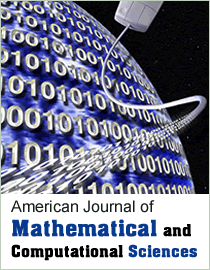IntroductionAmerican Journal of Mathematical and Computational Sciences is a peer-reviewed open access international journal, which is aimed to provide a publication forum for important research in different areas covering all aspects of mathematical and computational science, such as numerical analysis, optimization, linear and nonlinear programming, theory of computation, control theory, theory of algorithms, computational logic, applied combinatorics, coding theory, fuzzy theory, differential equations, algebra and number theory. The journal aims to publish original research papers, review papers and short communications, in all areas of mathematical and computational sciences. Submitted papers must be correct, original, nontrivial, and well written.

Aims and Scope

The journal welcomes the submission of manuscripts that meet the general criteria of significance and academic excellence.
Topics to be covered by this journal include, but are not limited to:

 Aggregation Functions Algebra Algebraic Geometry Algorithms and Data Structures Artificial Intelligence Calculus of Variations Combinatorics Complex Analysis Computational Science Computer Elements and System Architecture Computer Graphics Concurrent Database Management Dynamical Systems Eco-Epidemiology Epidemiology of Infectious Diseases Fluid Mechanics Formal Concept Analysis Functional Analysis Fuzzy Measures and Integrals Fuzzy Sets and Fuzzy Logic Geometry Group Theory Human-Computer Interactions Interval-Valued Fuzzy Sets Lie Algebras Logic Logic Programming Mathematical Analysis Mathematical Physics Number Theory Numerical Analysis Operational Research Optimal Control Ordinary Differential Equations Parallel and Distributed Systems Partial Differential Equations Population Dynamics Potential Theory Probability Theory Programming Languages and Compilers Rough Sets Scientific Computing Software Engineering Solid Mechanics Statistics Statistics and Game Theory Telecommunication and Networking Theoretical Computer Science Topology